# The Physics of a Falling Slinky

## Introduction

Using a simple household toy it is possible to observe a strange and intriguing physics phenomenon. If a slinky is hung by one end such that its own weight extends it, and that slinky is then released, the lower end of the slinky will not fall or rise, but will remain briefly suspended in air as though levitating.  To achieve the full visual effect, one may wish to attach a brightly colored object to the lower end of the slinky.  This visually impressive display can be modeled by treating the slinky as a tightly wound spring.  By applying Hooke's law in conjunction with Newton's second law, it is possible to solve for the static equilibrium of a hanging Slinky, and then use this result to solve for the motion of the Slinky as it falls. In particular, I will derive an expression for the "levitating time" over which the bottom of the Slinky remains motionless, and compare it to an experimentally measured value for a metal Slinky.

## The Hanging Slinky

A hanging Slinky may be treated as a hanging spring, but with a few qualifications. Real springs, including Slinkies, have coils of finite thicknesses, so that there is a minimum compressed length at which the coils are touching. Indeed, as opposed to an ideal spring, a Slinky is usually pretensioned, meaning that in the absence of an external force the coils are touching, and a finite force f = k (l1 - l0 ) is required to cause the coils to separate, where k is the spring constant, l1 is the minimum compressed length of the Slinky, and lo is the unachievable zero force length. 

To solve for the motion of a falling Slinky, we use a dimensionless variable d to denote points on the Slinky, where d = 0 denotes the top end of the slinky, and d =1 denotes the bottom. The difference in d between any two points on the slinky is equal to the fraction of the Slinky's total mass between those points. The position and motion of the Slinky is described by giving the location y(d,t) of all points d of the slinky as functions of time t.  For the hanging slinky, we find y0(d), which is static and not dependent on t. Above a certain point d1 the Slinky behaves like a loosely wound spring according to the equation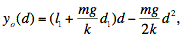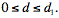(1)

Which is a direct result of Hooke's law.  Because the Slinky is pretensioned, below d1 the coils are in contact, because the weight of the Slinky below that point is not enough to overcome the finite force f. 

By setting d = d1 in equation 1, and adding l1 (1 - d1) (the length of the section below d1), we obtain the total suspended length of the slinky: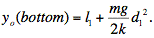(2)

Using equation 2, it is easy to experimentally determine the spring constant k of a Slinky by measuring its collapsed length l1, its suspended length yo(bottom), its mass m, and d1. I measured these values for a metal Slinky purchased at a local toy store in order to determine its spring constant k. My measurements are listed in Table 1. I obtained k = 0.80 ± 0.04 kg/s2 for the Slinky.

## The Falling Slinky

Having derived an expression for the static equilibrium of a hanging slinky, let us now suppose that at time t = 0 the top of the slinky is released. One coil after another begins to collapse down, with the coils below the leading edge of this collapse remaining motionless. The bottom of the Slinky appears to levitate in the air, remaining in the same spot until the leading edge of the collapsing portion of the slinky reaches d1, at which point the whole slinky falls to earth in a collapsed coil. I experimentally measured the "levitating time" of the metal Slinky, and found it to be t = 0.4 ± 0.1 seconds.

Table 1: Measurements for toy Slinky.
Mass m Suspended Length y0 Collapsed Length l1 Fraction Coiled d1
0.218 ± 0.001 kg 1.365 ± 0.003 m 0.057 ± 0.003 m 0.99 ± 0.005

As the Slinky falls, there are three portions of the slinky. The bottom portion, below d1, remains motionless and coiled together. The middle section, above d1 and below the leading edge of the collapse is yet to be affected by the fall and remains governed by equation 1. The locations of the points in the top portion, above the leading edge of the collapse, are given by: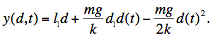(3)

Where d(t) is the location of the leading edge of the collapsed top section.  We get this result by realizing that the derivative of y(d,t) with respect to d for the top section must be l1 , because this section is collapsed, and from the boundary condition that y(d,t) for the top section must be equal to y(d,t)for the middle section at the point d=d(t).  If we take the derivative of equation 3 with respect to time, we get the velocity of the top collapsed section: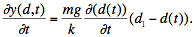(4)

The mass of the top portion of the slinky is given by md(t), and as this portion of the Slinky is the only portion in motion, the total momentum of the slinky is given by its mass times equation 4. Using Newton's second law, we can equate this momentum to the total impulse mgt that has acted on the system to get :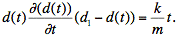(5)

By integrating equation 5 we get an equation for d(t):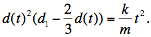(6)

Now, by plugging d(t) = d1 into equation 6, we find the time it takes until the Slinky collapses fully, and the bottom begins to move :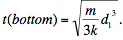(7)

Plugging the experimentally measured values for m and d1, and the value k calculated using equation 2, this gives a "levitating time" for the metal Slinky of t = 0.29 ± 0.05 seconds. This corresponds relatively well to the experimental value of t = 0.4 ± 0.1 seconds.

## Conclusion

When a Slinky is dropped, the bottom of the Slinky remains motionless as the top collapses towards it, making it appear to the observer as though the Slinky is levitating. By considering the Slinky as a tightly wound, pretensioned spring, the static equilibrium of a hanging Slinky was solved for using Hooke's law (Equation 1). This result was used to measure the spring constant of an actual metal Slinky. The motion of the Slinky after it is released at time t=0 was then solved for to derive an expression for the time over which the bottom of the Slinky remains motionless and the Slinky appears to levitate (Equation 7). This expression gave a value of t = 0.29 ± 0.05 seconds for the Slinky used in the experiments, which matches up very well with the experimentally measured value of t = 0.4 ± 0.1 seconds.

© 2007 Shimon Kolkowitz. The author grants permission to copy, distribute and display this work in unaltered form, with attribution to the author, for noncommercial purposes only. All other rights, including commercial rights, are reserved to the author.

## References

 Martin Gardner, "A Slinky Problem," Phys. Teach. 38, 78 (2000).

 Mark Graham, "Analysis of Slinky Levitation," Phys. Teach. 39, 90 ( 2001).

 M.G. Calkin, "Motion of a Falling Spring," Am. J. Phys. 61, 261 (1993).

 S. Y. Mak, "The Static Effectiveness Mass of a Slinky," Am. J. Phys. 55, 994 (1978).